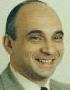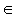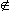# Cut The Knot!

An interactive column using Java applets
by Alex Bogomolny

# Morley's Redux and More

November 2000Happy is the man who knows to enjoy himself in things big and small. Here's the news: Alain Connes - a French Fields medalist - has come up with a new proof of Morley's theorem. Indeed the proof was published in 1998, and the news may be stale for some readers, but I was pleasantly surprised to learn about it as recently as last month. A message with the proof was posted to the sci.math.fr newsgroup and translated for the benefit of the Hyacinthos discussion group by its French member, Jean-Pierre Ehrmann. The group, thought up by John Conway and Antreas Hatzipolakis, was named after the French geometer Emile Michel Hyacinthe Lemoine (1840-1912). Its interests are appropriately centered around triangle geometry.

Connes was introduced to the theorem at lunch time. The theorem was mistakenly attributed to Napoleon Bonaparte (1769-1821) - another French geometer. Which, besides curiosity, served as an impetus to pick up the challenge. To remind, Morley's theorem states that

The three points of intersection of the adjacent trisectors of the angles of any triangle form an equilateral triangle.

Connes began with an observation that the points of intersection "are the fixed points of pairwise products of rotations gi around vertices of the triangle (with angles two thirds of the corresponding angles of the triangle)." Rotation is a transformation of the plane which is defined by the center of rotation - its fixed point - and the angle of rotation. The plane, and with it every figure in the plane, rotates around the center through the angle of rotation. The product of two rotations (which is often also called the sum) is the result of the successive execution of the two transformations. The product of two rotations is another rotation through an angle which is the sum of two rotation angles. If the angles add up to 0° (mod 360°), the product becomes a translation.

At first, Connes looked at gi's as plane isometries with the intention to express in their terms the three fold symmetry of the equilateral triangle. This attempt failed and eventually he established impossibility of such a presentation. The success came with the interpretation of gi's as affine transformations of the (complex) line.

The proof is carried out for a field k and the affine group G over k. G consists of affine transformations g(x) = ax + b, xk, where ak, a≠0 and bk. For g∈G, define

d(g) = d(ax + b) = ak*,

the multiplicative subgroup of non zero elements of k. d is a morphism from G to k*. Define also T = Ker(d), which is the group of translations, i.e. the additive subgroup of k. For gT, a≠1, and g admits exactly one fixed point:

fix(g) = b/(1 - a).

### Theorem

Let g1, g2, g3G be such that g1g2, g2g3, g3g1 and g1g2g3 are not in T. Let j = d(g1g2g3). The following two conditions are equivalent,

1. g13g23g33 = 1.
2. j3 = 1 and A + jB + j2C = 0, where A = fix(g1g2), B = fix(g2g3), C = fix(g3g1).

### Proof

Let gi = ai x + b, i = 1, 2, 3. The equality g13g23g33 = 1 is equivalent to d(g13g23g33) = 1, and b = 0, where b is the translational part of g13g23g33. The first condition is exactly j3 = 1. Also, by hypothesis j≠1. b is computed to be

b = (a12 + a1 + 1)b1 + a13(a22 + a2 + 1)b2 + (a1a2)3(a32 + a3 + 1)b3,

Using j = a1a2a3, we transform this into

b = -ja12a2(a1 - j)(a2 - j)(a3 - j)(A + jB + j2C),

where the fixed points have been expressed explicitly as

A = (a1b2 + b1)/(1 - a1a2), B = (a2b3 + b2)/(1 - a2a3), C = (a3b1 + b3)/(1 - a3a1).

Now, aij since by hypothesis the pairwise products of gi's are not translations. The theorem thus follows.

### Corollary (Morley's theorem)

Let there be given ΔPQR. Take k to be the field of complex numbers C and let g1 be the rotation with center P through two thirds of ∠QPR and similarly for g2 and g3.

g13g23g33 = 1 because each gi3 is the product of reflections in the two sides forming the corresponding angle. Due to the definition of rotation angles, A, B, and C are the intersections of trisectors. This establishes condition a) of the theorem, which implies b). In particular, A + jB + j2C = 0. The latter is a classic characterization of equilateral triangles.

### Remarks

1. I forgot to mention that there is no limitation on the characteristic of k, except that some complications arise when characteristic equals 3.

2. Expression g13g23g33 has a built-in invariance with respect to multiplication of any of gi's by a cubic root of 1. The theorem thus implies existence of the total of 18 equilateral triangles. As I mentioned on another occasion, these are often designated as Morley triangles. The fact is however that Morley has discovered 27 equilateral triangles which is clearly not reflected in the current nomenclature.

Connes published his proof in a collection of papers on the occasion of the 40th anniversary of l'Institut des Hautes Études Scientifiques. The volume addresses various aspects of the evolution of the relationship between mathematics and physics. Most of the papers are historical surveys with emphasis on the last few decades. Symmetry groups are of course only one element in the multifaceted relationship. Topology, cohomology, Lie algebras, knot theory have all become valuable items in the physicist' tool chest. More than that, with the emergence of the superstring theory, a theory that holds a promise of becoming a TOE - a Theory Of Everything, the attitude of physicist towards mathematics underwent a definite metamorphosis.

L. Michel who joined l'IHES in 1962 writes in Physique et Mathématique: Mais je n'y ai été qu'un utilisateur, très gourmand et un peu gourmet, de mathématiques ... G. Veneziano recollects how in the early 1960s Prof. Gatto was used to say: A good theorist should not study maths, should just know it! But he ends up his article Physics And Mathematics: A Happily Evolving Marriage? with a remark that "... mathematical rigour and elegance are becoming a driving force, a true guiding principle for theoretical research" and adds a dictum of his own:

Let us invent
tools that suit our problems
rather than
problems that suit our tools.

Yuval Ne'eman of Tel-Aviv University, Israel makes a reference to the Erlangen Program announced by Felix Klein in 1872. Klein suggested to classify different geometries by means of groups of symmetries - transformations that leave invariant essential properties of geometric figures. In his paper De l'Autogéométrisation de la Physique, Ne'eman writes: Le Programme d'Erlangen se réalise à travers la physique! (Well, at times symmetry gets spontaneously lost and a definitive reference about this phenomenon is Noncommutative Geometry by Alain Connes.)

So physicists turn into mathematicians. In fact, this may not be at all surprising. V.I. Arnold has once categorized mathematics as that part of physics in which experiments are inexpensive. Given the growing costs of experimentation in particle physics, the evolution of theoretical physics into a part of mathematics might appear as a natural development.

Arnold made his remark during a discussion on mathematics education that took place at Palais de Découverte in 1997. The thrust of his argument was directed against what is known in the United States as the new math, although his outlook was much broader. He lamented an attempt that originated in France in the middle of the 20th century to separate mathematics from physics, in mathematics instruction in particular. The attempt is associated with a group of (then) young mathematicians that published volumes of mathematical texts in a rigorous axiomatic manner under the assumed name of N. Bourbaki.

Incidentally, J. Dieudonné - one of the more active contributors in the group - was one of the first two mathematics professors (the other one was Alexander Grothendieck) who received a permanent appointment at l'IHES in 1959. Bourbaki's treatise was extremely influential on the development of mathematics and physics - and not necessarily in a negative sense. Here's just one short affirmation from the aforementioned collection of papers: Bourbaki traite l'intégrale de Wiener d'une manière que je pouvais généraliser facilement à l'intégrale de Feynman (C. Dewitt-Morette, Des Ponts).

As regard mathematics education, Arnold holds a two-fold view. First, he classifies mathematics as a natural science. Elsewhere (Polymathematics: is mathematics a single science or a set of arts?) Arnold writes:

All mathematics is divided into three parts: cryptography (paid for by CIA, KGB and the like), hydrodynamics (supported by manufacturers of atomic submarines) and celestial mechanics (financed by military and other institutions dealing with missiles, such as NASA).

Cryptography has generated number theory, algebraic geometry over finite fields, algebra, combinatorics and computers.

Hydrodynamics procreated complex analysis, partial derivative equations, Lie groups and algebra theory, cohomology theory and scientific computing.

Celestial mechanics is the origin of dynamical systems, linear algebra, topology, variational calculus and symplectic geometry.

The existence of mysterious relations between all this different domains is the most striking and delightful feature of mathematics (having no rational explanation).

Second, as every natural science, mathematics is being developed by trial and error, modeling and experimentation, with proofs coming last as a method of hypothesis verification. Both aspects of mathematics must be reflected in mathematics education.

In fact the trend of melding of theoretical physics with mathematics was reciprocated on the part of mathematics by fostering its experimental nature. Technology (besides traditional paper and pencil) permeates today's mathematics. Triangle geometry serves as a good example.

Clark Kimberling - the man largely responsible for a renewed interest in triangle geometry - writes

Euclid's Elements and other remnants of ancient Greek times contain theorems about triangles and Descriptions of four triangle centers: centroid, incenter, circumcenter, and orthocenter.

Later triangle geometers include Euler, Pascal, Ceva, and Feuerbach. In 1873, Emile Lemoine presented a paper "on a remarkable point of the triangle," now known as the Lemoine point or symmedian point.

...

A huge wave of interest and publications in triangle geometry swept through the last years of the 19th century but then collapsed during the early years of the 20th.

Kimberling's book Triangle Centers and Central Triangles published in 1998 describes nearly 400 various centers and his online encyclopedia currently lists in excess of 500 remarkable points. (Points X356 and X357 are associated with Morley's triangle and are known as the 1st and 2nd Morley centers.) Most of these were first discovered with the help of computer.

Computers open new horizons for experimentation while still keeping experiments inexpensive. As a tool in mathematics education, computers hold a promise to get more students involved in mathematical experimentation. It remains to be seen whether many will and with what results. Experimentation leads to uncertainty. No doubt many students will find this difficult. New pedagogy will be required to teach how to use computers successfully. Meanwhile, I wish to offer a curious example. I borrowed the example from Paul Yiu's lecture at the 7th Statewide Conference organized by Florida Higher Education Consortium, November 12, 1998.

Let I be the incenter of ΔABC. Consider three triangles AIB, BIC, and CIA. In each of the three triangles pass a straight line through its incenter and circumcenter. (In the applet, IC and OC stand for the incenter and circumcenter of ΔAIB, and similarly for the other 4 points.) Doodling with the applet one may form (at least) two conjectures. (A working geometer would probably dismiss one of them as a known result. I still carry on.)

1. Circumcenters of triangles AIB, BIC, and CIA all lie on the circumcircle of triangle ABC.
2. The three lines through the incircle and the circumcircle of triangles AIB, BIC, and CIA meet at a point.

In the applet, by checking the Show calculations you may obtain the (approximate) sum of the Distances of the circumcenters OA, OB, OC to the circumcircle of ΔABC and the (approximate) Area of the triangle formed by the above three lines.

### This applet requires Sun's Java VM 2 which your browser may perceive as a popup. Which it is not. If you want to see the applet work, visit Sun's website at https://www.java.com/en/download/index.jsp, download and install Java VM and enjoy the applet.

You may see that one conjecture is probably right whereas the other conjecture is probably wrong. You may want to formally prove the former. There is no disagreement between mathematicians that a proof should crown any kind of purposeful experimentation. According to Floor van Lamoen - a Dutch member of the Hyacinthos group - "computer exploration is nice. Proving is even nicer!"

And just one more note. Paul Yiu is one of the organizers of a new online publication: Forum Geometricorum. This is a peer reviewed journal devoted to the Euclidean geometry. The first issue planned for January 2001.

### References

1. Les Relations Entre Les Mathématiques et La Physique Théorique, Institut des Hautes Études Scientifiques, 1998
2. I. M. Yaglom, Geometric Transformations, v 1, MAA, 1962### Morley's Miracle

#### Algebraic proofs

1. Morley's Redux and More

#### Invalid proofs